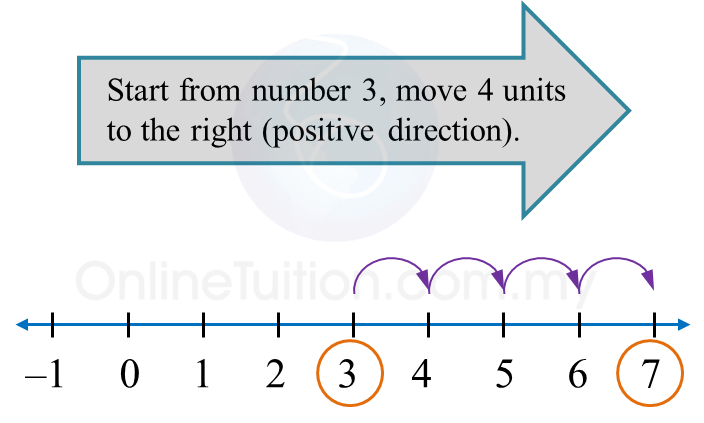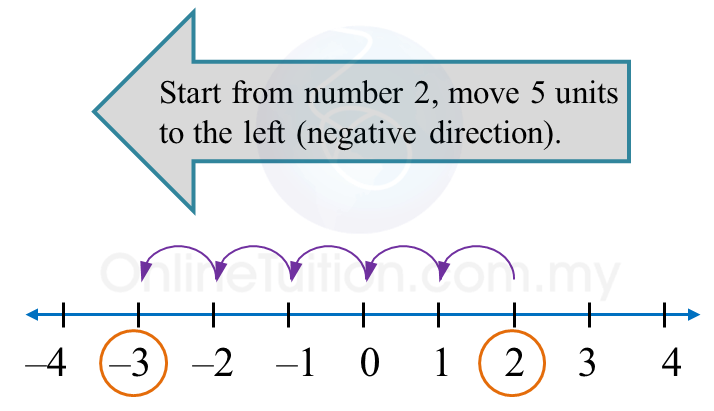# 1.1.1 Integers

1.1.1 Addition and Subtraction of Integers

1.
Addition of integers can be done on a number line.
(a) To add a positive integer:
Move to the right (positive direction) on the number line.

(b)
Move to the left (negative direction) on the number line.2. Integers
with the same signs are called integers with like signs.
Example:
2 and 7, –25 and –5.

3. Integers with the different signs are called integers with unlike signs.
Example:
+2 and –7, –25 and 5.

Example 1:

Solve the following.
(a) 3 + (+4)
(b) 2 + (–5)

Solution:
(a)Therefore,
3 + (+4) = 3 + 4
= 7

(b)Therefore,
2 + (–5)
= –3

1.1.1b Multiplication and Division of Integers
1. Multiplication and division of like signs gives (+)

$\overline{)\begin{array}{l}\text{}\left(+\right)×\left(+\right)=+\text{}\left(+\right)÷\left(+\right)=+\text{}\\ \text{}\left(-\right)×\left(-\right)=+\text{}\left(-\right)÷\left(-\right)=+\end{array}}$

2.
Multiplication and division of unlike signs gives ()

$\overline{)\begin{array}{l}\text{}\left(+\right)×\left(-\right)=-\text{}\left(+\right)÷\left(-\right)=-\text{}\\ \text{}\left(-\right)×\left(+\right)=-\text{}\left(-\right)÷\left(+\right)=-\end{array}}$

Example:
(a) –25 ÷ 5 = –5
(b)   8 × (–5) = –40